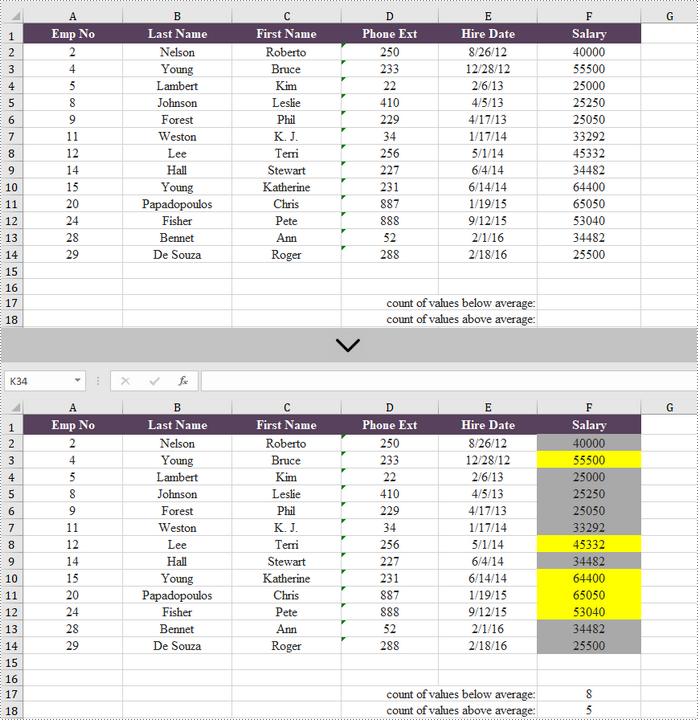# Java: Highlight Values Above or Below Average in Excel

If you have a lot of data in Excel, finding the special values can be challenging. In this situation, you can use the conditional formatting to automatically highlight the cells that contain the value that meets certain criteria. This article introduces how to highlight values that are above or below the average using conditional formatting in Java, using Spire.XLS for Java.

### Install Spire.XLS for Java

First, you're required to add the Spire.Xls.jar file as a dependency in your Java program. The JAR file can be downloaded from this link. If you use Maven, you can easily import the JAR file in your application by adding the following code to your project's pom.xml file.

```<repositories>
<repository>
<id>com.e-iceblue</id>
<name>e-iceblue</name>
<url>https://repo.e-iceblue.com/nexus/content/groups/public/</url>
</repository>
</repositories>
<dependencies>
<dependency>
<groupId>e-iceblue</groupId>
<artifactId>spire.xls</artifactId>
<version>13.11.6</version>
</dependency>
</dependencies>
```

### Highlight Values Above or Below Average Excel in Java

Below are the steps to highlight values above or below average in Excel using Spire.XLS for Java.

• Create a Workbook object.
• Get a specific worksheet from the workbook using Workbook.getWorksheets.get(index) method.
• Add a conditional formatting to the worksheet using Worksheet.getConditionalFormats().add() method and return an object of XlsConditionalFormats class.
• Set the cell range where the conditional formatting will be applied using XlsConditionalFormats.AddRange() method.
• Add an average condition using XlsConditionalFormats.addAverageCondition() method, specify the average type to above and change the background color of the cells that meet the condition to yellow.
• Add another average condition to change the background color of the cells that contain the value below average to light gray.
• Save the workbook to an Excel file using Workbook.saveToFile() method.
• Java
```import com.spire.xls.AverageType;
import com.spire.xls.ExcelVersion;
import com.spire.xls.Workbook;
import com.spire.xls.Worksheet;
import com.spire.xls.core.IConditionalFormat;

import java.awt.*;

public class HighlightValuesAboveAndBelowAverage {

public static void main(String[] args) {

//Create a Workbook object
Workbook workbook = new Workbook();

//Get the first worksheet
Worksheet sheet = workbook.getWorksheets().get(0);

//Add a conditional format to the worksheet

//Set the range where the conditional format will be applied

//Add a condition to highlight values above average with yellow
condition1.setBackColor(Color.yellow);

//Add a condition to highlight values below average with light gray
condition2.setBackColor(Color.lightGray);

//Get the count of values below average
sheet.getRange().get("F17").setFormula("=COUNTIF(F2:F14,\"<\"&AVERAGE(F2:F14))");

//Get the count of values above average
sheet.getRange().get("F18").setFormula("=COUNTIF(F2:F14,\">\"&AVERAGE(F2:F14))");

//Save the workbook to an Excel file
workbook.saveToFile("output/HighlightValues.xlsx", ExcelVersion.Version2016);
}
}```### Apply for a Temporary License

If you'd like to remove the evaluation message from the generated documents, or to get rid of the function limitations, please request a 30-day trial license for yourself.